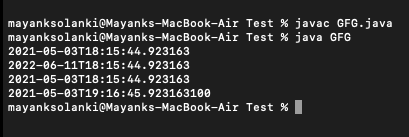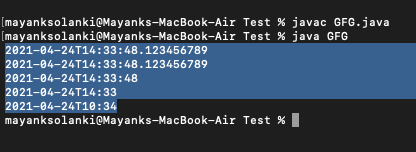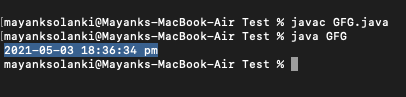# java.time.LocalDateTime Class in Java

• Last Updated : 26 May, 2021

java.time.LocalDateTime class, introduced in Java 8, represents a local date-time object without timezone information. The LocalDateTime class in Java is an immutable date-time object that represents a date in the yyyy-MM-dd-HH-mm-ss.zzz format. It implements the ChronoLocalDateTime interface and inherits the object class.

Wherever we need to represent time without a timezone reference, we can use the LocalDateTime instances. LocalDateTime, for example, can be used to start batch jobs in any application. Jobs will be run at a fixed time in the timezone in which the server is located. Note LocalDateTime instances are immutable and thread.

Syntax: Class declaration

public final class LocalDateTime

extends Object

Methods of this class are as follows:

Some more methods to modify local time are as follows  in LocalDateTime can be used to get to a new localdatetime instance relative to an existing localdatetime instance. They are namely as follows:

plusYears(), plusMonths(), plusDays(), plusHours(), plusMinutes(), plusSeconds(), plusNanos(), minusYears(), minusMonths(), minusDays(), minusHours(), minusMinutes(), minusSeconds(), minusNanos()

Examples 1:

## Java

 `// Java Program to illustrate LocalDateTime Class of java.time package  `   `// Importing LocalDateTime class from java.time package` `import` `java.time.LocalDateTime;`   `// Main class for LocalDateTime` `public` `class` `GFG {`   `    ``// Main driver method` `    ``public` `static` `void` `main(String[] args)` `    ``{` `        ``// Creating an object of LocalDateTime class` `        ``// in the main() method` `        ``LocalDateTime now = LocalDateTime.now();`   `        ``// Print statement` `        ``System.out.println(now);`   `        ``// Adding 1 year, 1 month, 1 week and 1 day` `        ``LocalDateTime localDateTime1 = now.plusYears(``1``)` `                                           ``.plusMonths(``1``)` `                                           ``.plusWeeks(``1``)` `                                           ``.plusDays(``1``);` `        ``// Print statement` `        ``System.out.println(localDateTime1);`   `        ``// Subtracting 1 year, 1 month, 1 week and 1 day` `        ``LocalDateTime localDateTime2` `            ``= localDateTime1.minusYears(``1``)` `                  ``.minusMonths(``1``)` `                  ``.minusWeeks(``1``)` `                  ``.minusDays(``1``);` `        ``// Print statement` `        ``System.out.println(localDateTime2);`   `        ``// Adding 1 hour, 1 minute, 1 second and 100` `        ``// nanoseconds` `        ``LocalDateTime localDateTime3` `            ``= localDateTime2.plusHours(``1``)` `                  ``.plusMinutes(``1``)` `                  ``.plusSeconds(``1``)` `                  ``.plusNanos(``100``);` `        ``// Print statement` `        ``System.out.println(localDateTime3);`   `        ``// Subtracting 1 hour, 1 minute, 1 second and 100` `        ``// nanoseconds` `        ``LocalDateTime localDateTime4` `            ``= localDateTime3.minusHours(``1``)` `                  ``.minusMinutes(``1``)` `                  ``.minusSeconds(``1``)` `                  ``.minusNanos(``100``);` `        ``// Print statement` `        ``System.out.println(localDateTime4);` `    ``}` `}`

Output:Example 2: Creating a specified time

## Java

 `// Java Program to illustrate LocalDateTime Class` `// of java.time package by creating specific time `   `// Importing required classes from resp packages ` `import` `java.time.*;` `import` `java.time.format.*;`   `// main class ` `class` `GFG {`   `    ``// Main driver method` `    ``public` `static` `void` `main(String[] args)` `    ``{`   `        ``// Milliseconds` `        ``LocalDateTime localDateTime1 = LocalDateTime.of(` `            ``2021``, ``04``, ``24``, ``14``, ``33``, ``48``, ``123456789``);` `        ``// Print statement` `        ``System.out.println(localDateTime1);`   `        ``// Month` `        ``LocalDateTime localDateTime2 = LocalDateTime.of(` `            ``2021``, Month.APRIL, ``24``, ``14``, ``33``, ``48``, ``123456789``);` `        ``// Print statement` `        ``System.out.println(localDateTime2);`   `        ``// Seconds` `        ``LocalDateTime localDateTime3 = LocalDateTime.of(` `            ``2021``, Month.APRIL, ``24``, ``14``, ``33``, ``48``);` `        ``// Print statement` `        ``System.out.println(localDateTime3);`   `        ``// Minutes` `        ``LocalDateTime localDateTime4 = LocalDateTime.of(` `            ``2021``, Month.APRIL, ``24``, ``14``, ``33``);` `        ``// Print statement` `        ``System.out.println(localDateTime4);`   `        ``// Local date + Local time` `        ``LocalDate date = LocalDate.of(``2021``, ``04``, ``24``);` `        ``LocalTime time = LocalTime.of(``10``, ``34``);`   `        ``LocalDateTime localDateTime5` `            ``= LocalDateTime.of(date, time);` `        ``// Print statement` `        ``System.out.println(localDateTime5);` `    ``}` `}`

Output:Example 3: Format LocalDateTime to string

To format a local time to the desired string representation, use the LocalDateTime.format(DateTimeFormatter) method.

## Java

 `// Java Program to illustrate LocalDateTime Class by` `// Formatting LocalDateTime to string`   `// Importing all classes from java.time package` `import` `java.time.LocalDateTime;` `import` `java.time.format.*;` `import` `java.util.*;`   `// Main class` `class` `GFG {`   `    ``// Main driver method` `    ``public` `static` `void` `main(String[] args)` `    ``{`   `        ``// Creating an object of DateTimeFormatter class` `        ``DateTimeFormatter formatter` `            ``= DateTimeFormatter.ofPattern(` `                ``"yyyy-MM-dd HH:mm:ss a"``);`   `        ``// Creating an object of LocalDateTime class` `        ``// and getting local date and time using now()` `        ``// method` `        ``LocalDateTime now = LocalDateTime.now();`   `        ``// Formatting LocalDateTime to string` `        ``String dateTimeString = now.format(formatter);`   `        ``// Print and Display` `        ``System.out.println(dateTimeString);` `    ``}` `}`

Output:Note: In order to parse a string to LocalDateTime, convert time in a string to a local time instance, the LocalDateTime class has two overloaded parse() methods.

• parse(CharSequence text)
• parse(CharSequence text, DateTimeFormatter formatter)

My Personal Notes arrow_drop_up
Recommended Articles
Page :